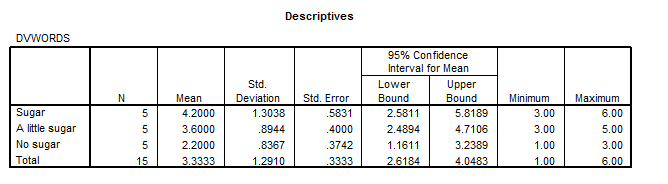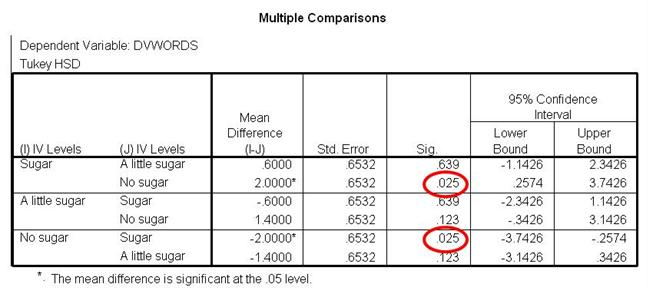How do I interpret data in SPSS for a 1-way between subjects ANOVA?

Home > How do I interpret data in SPSS for a 1-way between subjects ANOVA?

Background | Enter Data | Analyze Data | Interpret Data | Report Data

## Descriptives Box

Take a look at this box. You can see each condition name in left most column. If you have given your conditions meaningful names, you should know exactly which conditions these names represent. You can find out the number of participants, the mean and standard deviation for each condition by reading across each of the three condition rows. You can also see things like the minimum and maximum value in each condition, as well as confidence intervals and standard error.## Example

In this Descriptive Statistics box, the mean for the sugar condition is 4.20. The mean the mean for the a little sugar condition is 3.60 and the mean for the no sugar condition is 2.20. The standard deviation for the sugar condition is 1.30, the standard deviation for the a little sugar condition is 0.89 and the standard deviation for the no sugar condition is 0.84 (when rounded).  The number of participants in each condition (N) is 5.

## Compare the Means

We use ANOVA to determine if the means are statistically different. But you don’t have to use ANOVA to find out some basic information about mean differences. Compare your means. Which one is the highest? Which is the lowest? If you were to find significant differences with your ANOVA, what do these directional differences in the means say about your results? In this example, the mean for the sugar condition is 4.2 words whereas the mean for the no sugar condition is 2.2 words remembered. The mean for the A little Sugar Condition, 3.6, falls in between these two. So just eyeballing it, we can see that there are more words remembered in the Sugar condition. We need our ANOVA to determine if the differences between condition means are significant. We need ANOVA to make a conclusion about whether the IV (sugar amount) had an effect on the DV (number of words remembered). But looking at the means can give us a head start in interpretation.

## ANOVA Box

This is the next box you will look at. It shows the results of the 1 Way Between Subjects ANOVA that you conducted. Take a loot at the Sig. value in the last column.## Sig value

This value will help you determine if your condition means were relatively the same or if they were significantly different from one another. Put differently, this value will help you determine if your IV had an effect. In this example, the Sig. value is 0.027.

## If the Sig value is greater than 05…

You can conclude that there is no statistically significant difference between your three conditions. You can conclude that the differences between condition Means are likely due to chance and not likely due to the IV manipulation.

If the Sig value is less than or equal to .05…

You can conclude that there is a statistically significant difference between your three conditions. You can conclude that the differences between condition Means are not likely due to change and are probably due to the IV manipulation.

## Our example

The Sig. value in our example is 0.027. This value is less than .05. Because of this, we can conclude that there is a statistically significant difference between the mean number o words remembered for all of our conditions (sugar, a little sugar, no sugar).

## The problem

The Sig value does not tell you which condition means are different. It could be that only the sugar condition is significantly different than the no sugar condition in terms of number of words remembered. It could be that only the a little sugar condition is significantly different than the no sugar condition. It could be that all conditions are significantly different from each other. The Sig value can tell us that there is a significant difference between some of the conditions. It just cannot tell us which ones.

## The solution

Researchers have solved this problem by conducting post hoc tests. These tests are used when he have found statistical significance between conditions but when we don’t know where the significant differences are. These tests are not used when the results of a 1-Way Between Subjects ANOVA are not significant because there is no need. But when we do find a statistically significant result, when the Sig. value is less than .05, we need to use these tests.

## Types of tests to use

When you analyzed your data, you might remember being asked to click on the Post Hoc button. This button brought up a Post Hoc Multiple Comparisons box with many options to check. These options represented various post hoc tests. Most of these tests have strange names (like Bonferroni and Scheffe) but that’s just because they are named after the people who invented them. You were asked to check two tests, Tukey and Dunnett’s T3. You really could have checked as many as you liked. The Tukey test is popular so we will focus on that one. If you find a significant result with a 1-Way Between Subjects ANOVA, and if your IV has 3 levels, you will need to use the results of a post hoc test like the Tukey test to compare

ü     Condition 1 and Condition 2

ü     Condition 1 and Condition 3

ü     Condition 2 and Condition 3

## Our cxample

Because of the fact that we found a statistically significant result in our example, we would want to look at the results of a post hoc test like Tukey. This will help us find out which of our conditions were significantly different from each other. We conduct post hoc tests like the Tukey to compare each of the following conditions.

ü      Sugar and No Sugar

ü      A little Sugar and No Sugar

ü      A little Sugar and Sugar

# Multiple comparisons box

This is the place where you will look to find the results of your post hoc tests. In the first column of this box, you will see your condition names. The condition names appear in six rows. These rows show the comparisons of various conditions. On the left hand side of every other row, you will see a single condition name. On the right hand side of each row, you will see a condition name. The statistics that you see in the columns to the right of the first column show you the comparison between the condition name on the left and each of the condition names on the right.## Our example

In our example, the condition name Sugar appears in the first column on the left hand side of the top row. The condition name A little sugar appears to the right of it. If we read across this particular row, we will see statistics that compare Sugar and A little sugar conditions. We can also see the name No Sugar in the first column on the right hand side of the second row. If we read across this particular row, we see statistics that compare Sugar and No Sugar conditions.

## The Sig value

Take a look at the Sig value when reading across each row. The Sig. value will tell you whether the two conditions that are being compared are significantly different. If the conditions are significantly different, the Mean Difference value in the corresponding row will also contain a star (*). There is more that one way to determine significance when using this type of post hoc test.

## If the Sig value is greater than 05…

You can conclude that there is no statistically significant difference between the two conditions that are being compared. You can conclude that the differences between condition Means are likely due to chance and not likely due to the IV manipulation.

If the Sig value is less than or equal to .05…

You can conclude that there is a statistically significant difference between the two conditions being compared. You can conclude that the differences between condition Means are not likely due to chance and are probably due to the IV manipulation.

## Our Example

Looking at the Sig. column in our example, we can see that most of the values are greater than .05. However, there are two values that are 0.025. These values correspond with the comparison between the Sugar and No Sugar conditions. For this reason, we can conclude that the Sugar and No Sugar conditions are significantly different in terms of words remembered. However, the other condition comparisons are not significantly different from one another. This means that the A little Sugar condition and No Sugar condition are not significantly different. It also means that the A little Sugar condition and Sugar condition are not significantly different.

Background | Enter Data | Analyze Data | Interpret Data | Report Data

Home | Copyright 2008 | Tell a Friend